1. Home

 Key Genstat Expression Mathematical expression Notes DescriptionC = A + B ci = ai + bi Element-wise additionC = A − B ci = ai − bi Element-wise subtractionC = A * B ci = ai × bi 1 Element-wise multiplicationC = A / B ci = ai / bi Element-wise division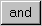C = A .AND. B ci = ai ∧ bi 2,3 Element-wise AND operation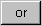C = A .OR. B ci = ai ∨ bi 2,4 Element-wise OR operationC = A .EQS. B ci = 1.0 if ai = bi ci = 0.0 if ai ≠ bi 5 Element-wise string equality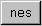C = A .NES. B ci = 0.0 if ai = bi ci = 1.0 if ai ≠ bi 5 Element-wise string inequalityC = A ** B ci = aibi Element-wise exponentiationC = A *+ B C = AB 6 Matrix product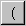( ( Left bracket. Used to control order of evaluation. Can be nested as many times as required) ) Right bracket.C = A == B ci = 1.0 if ai = bi ci = 0.0 if ai ≠ bi Element-wise test of equality. Can also be typed in as .EQ.C = A /= B ci = 1.0 if ai ≠ bi ci = 0.0 if ai = bi Element-wise test of inequality. Can also be typed in as .NE.C = A < B ci = 1.0 if ai < bi ci = 0.0 if ai ≥ bi Element-wise less than. Can also be typed in as .LT.C = A <= B ci = 1.0 if ai ≤ bi ci = 0.0 if ai > bi Element-wise less than or equal to. Can also be typed in as .LE.C = A > B ci = 1.0 if ai > bi ci = 0.0 if ai ≤ bi Element-wise greater than. Can also be typed in as .GT.C = A >= B ci = 1.0 if ai ≥ bi ci = 0.0 if ai < bi Element-wise greater than or equal to. Can also be typed in as .GE.C = A .IN. B ci = 1.0 if ai ∈ B ci = 0.0 if ai ∉ bi 7 Element-wise test of set inclusionC = A .NI. B ci = 1.0 if ai ∈ B ci = 0.0 if ai ∉ bi 7 Element-wise test of set non-inclusion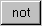B = NOT(A) bi = ¬ ai Element-wise logical negation (NOT).C = A .IS. B 8,9 Logical test of equivalence of identifiers. In the calculation C=A.IS.B the result C is 1 if A and B are identifiers of the same data structure, after any necessary substitutions (for example if B is a dummy in a FOR loop). The result is 0 if the identifiers are different.C = A .ISNT. B 8,9 Logical test of non-equivalence of identifiersC = A .EOR. B Element-wise exclusive OR operation